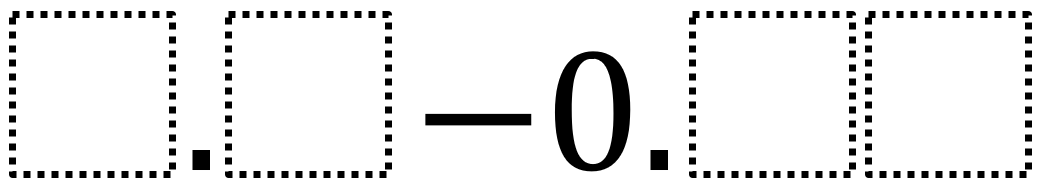# Subtracting Decimals to Make Them As Close to One as Possible

Directions: Use the digits 1 to 9, at most one time each, to fill in the boxes to get the difference that is as close to 1 as possible.### Hint

What digit should go in the ones place?

1.3 – 0.29
1.4 – 0.39
1.5 – 0.49
1.6 – 0.59
1.7 – 0.69
1.8 – 0.79

Source: Giselle Garcia

## Equivalent Expressions with Fractions

Directions: Using the digits 0 to 9 at most one time each and choosing either …

1.What about 1.2 – 0.21 = 0.99?

•Never telling my name.

“1.2 – 0.21 = 0.99” has two 9’s.

2.•There are two nines and you can’t use zeros

3.1.1-0.09=1.01
1-0.02=0.98

•In the first one there are two ones and for the second one you can’t use zeros

4.1.2 -0.21 = 0.99

5.This is my corrected answer : 1.6 – 0.58 = 1.02

•Nice, but, why wouldn’t you use 0.59? Am I missing something?

6.7.1.2-0.34=0.86

1.30-0.24=1.06

1.90-0.87=1.03

My last drawing is my answer this was hard!

8.I put 1.2 – 0.21 =0.99

9.1.3-0.42=0.88

10.1.5 – .49

11.1.7- 0.7
3.9-2.9

12.1.30-0.30

13.i aint telling my name

1.6 – 0.58 = 1.02